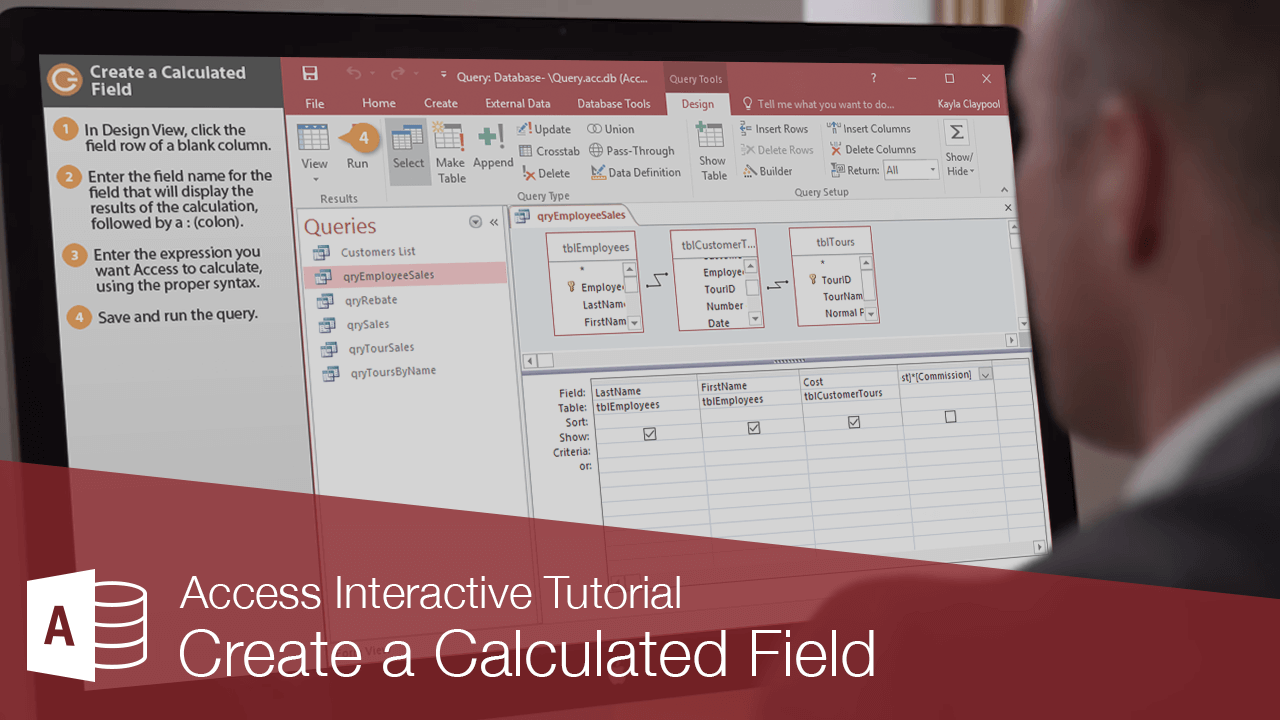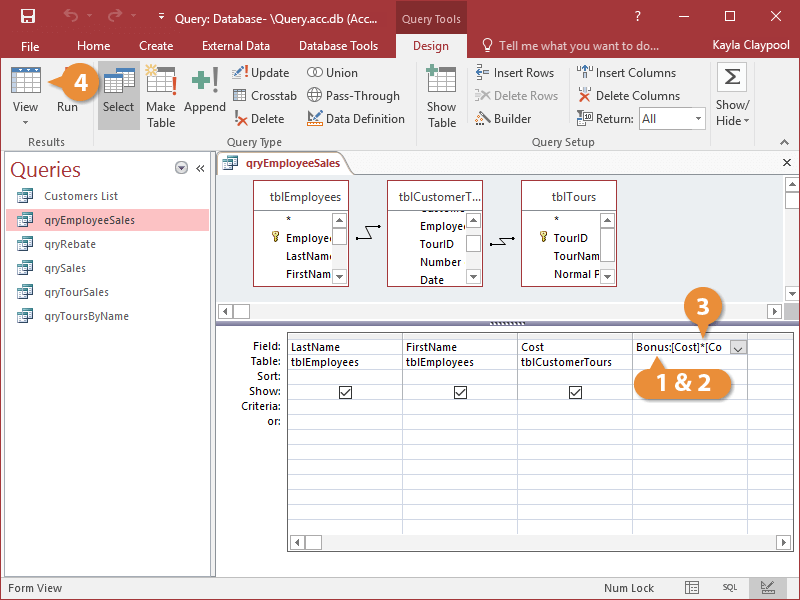Create a Calculated Field | CustomGuide# Create a Calculated Field

How to Create a Calculated Field in AccessA calculated field performs some type of arithmetic on one or more fields in a database to come up with a completely new field.

For example, you could calculate the sales tax for an invoice by multiplying an invoice total field by a tax rate. You must create an expression to perform a calculation. To enter fields in an expression, type the field name in brackets. If a field name exists in more than one table, you need to specify the field's table in brackets, followed by an exclamation point.

1. In Design View, click the field row of a blank column in the design grid.
2. Enter the field name for the field that will display the results of the calculation, followed by a colon (:).
3. Enter the expression you want Access to calculate, using the proper syntax.
4. For example, the expression Bonus:[Cost]*[Commission] will create a new calculated field named Bonus that will display the results of the Cost field from one table multiplied by the Commission field from another table.

Use arithmetic operators such as multiplication (*), addition (+), subtraction (-), division (/), and exponentiation (^) to create your expressions.

5. Save and run the query.Access displays the results of the query.